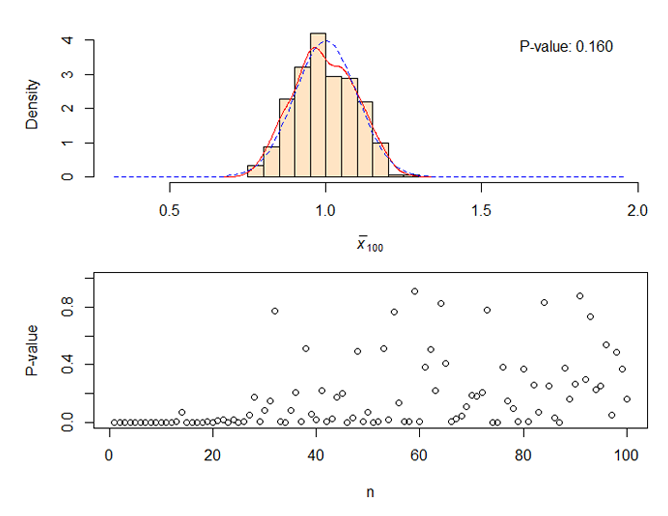• Posts tagged "概率"

# Blog Archives

## 概率基础和R语言

R的极客理想系列文章，涵盖了R的思想，使用，工具，创新等的一系列要点，以我个人的学习和体验去诠释R的强大。

R语言作为统计学一门语言，一直在小众领域闪耀着光芒。直到大数据的爆发，R语言变成了一门炙手可热的数据分析的利器。随着越来越多的工程背景的人的加入，R语言的社区在迅速扩大成长。现在已不仅仅是统计领域，教育，银行，电商，互联网….都在使用R语言。

• 张丹(Conan), 程序员Java,R,PHP,Javascript
• weibo：@Conan_Z
• blog: http://blog.fens.me
• email: bsspirit@gmail.comR语言是统计语言，概率又是统计的基础，所以可以想到，R语言必然要从底层API上提供完整、方便、易用的概率计算的函数。让R语言帮我们学好概率的基础课。

1. 随机变量
2. 随机变量的数字特征
3. 极限定理

## 1. 随机变量

• 什么是随机变量？
• 离散型随机变量
• 连续型随机变量

1). 什么是随机变量？

R程序：生成一个在(0,1,2,3,4,5)的随机变量

``````
> S<-1:5
> sample(S,1)
 2
> sample(S,1)
 3
> sample(S,1)
 5
``````

2). 离散型随机变量

R程序：生成样本空间为(1,2,3)的随机变量X，X的取值是有限的

``````
> S<-1:3
> X<-sample(S,1);X
 2
``````

3). 连续型随机变量

R程序：生成样本在空间(0,1)的连续随机函数，取10个值

``````
> runif(10,0,1)
 0.3819569 0.7609549 0.6692581 0.6314708 0.5552201 0.8225527 0.7633086 0.4667188 0.1883553
 0.3741653
``````

## 2. 随机变量的数字特征

• 数学期望
• 方差
• 标准差
• 各种分步的期望和方差
• 常用统计量(最大,最小,中位数,四分位数)
• 协方差
• 相关系数
• 矩(原点矩,中心矩,偏度,峰度)
• 协方差矩阵

1). 数学期望(mathematical expectation)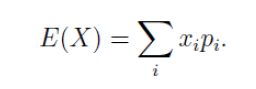R程序：计算样本(1,2,3,7,21)的数学期望

``````
> S<-c(1,2,3,7,21)
> mean(S)
 6.8
``````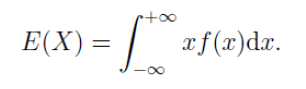2). 方差(Variance)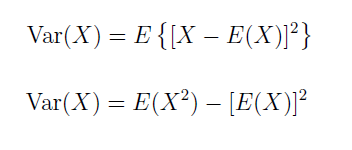R程序：计算样本(1,2,3,7,21)的方差

``````
> S<-c(1,2,3,7,21)
> var(S)
 68.2
``````

3). 标准差(Standard Deviation)

R程序：计算样本(1,2,3,7,21)标准差

``````
> S<-c(1,2,3,7,21)
> sd(S)
 8.258329
``````

4). 各种分步的期望和方差

• 离散型分布：两点分布，二项分布，泊松分布等
• 连续型分布：均匀分布，指数分布，正态分布，伽马分布等

5). 常用统计量

R程序：计算样本(1,2,3,3,3,7,7,7,7,9,10,21)的众数

``````
> S<-c(1,2,3,3,3,7,7,7,7,9,10,21)
> names(which.max(table(S)))
 "7"
``````

R程序：计算样本(2,3,3,3,7,7,7,7,9,10,21)的最小值

``````
> S<-c(2,3,3,3,7,7,7,7,9,10,21)
#最小值
> min(S)
 2
#最小值的索引
> which.min(S)
 1
``````

R程序：计算样本(2,3,3,3,7,7,7,7,9,10,21)的最大值

``````
> S<-c(2,3,3,3,7,7,7,7,9,10,21)
#最大值
> max(S)
 21
#最大值的索引
> which.max(S)
 11
``````

R程序：计算样本(1,2,3,4,5)的中位数

``````
> S<-c(1,2,3,4,5)
> median(S)
 3
``````

R程序：计算样本(1,2,3,4,5,6,7,8,9)的四分位数

``````
> S<-c(1,2,3,4,5,6,7,8,9)
> quantile(S)
0%  25%  50%  75% 100%
1    3    5    7    9
> fivenum(S)
 1 3 5 7 9
``````

R程序：计算样本(1,2,3,4,5,6,7,8,9)的统计函数

``````
> S<-c(1,2,3,4,5,6,7,8,9)
> summary(S)
Min. 1st Qu.  Median    Mean 3rd Qu.    Max.
1       3       5       5       7       9
``````

6). 协方差(Covariance)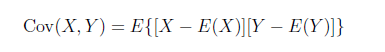R程序：计算X(1,2,3,4)和Y(5,6,7,8)的协方差

``````
> X<-c(1,2,3,4)
> Y<-c(5,6,7,8)
> cov(X,Y)
 1.666667
``````

7). 相关系数(Correlation coefficient)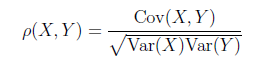R程序：计算X(1,2,3,4)和Y(5,7,8,9)的相关系数

``````
> X<-c(1,2,3,4)
> Y<-c(5,7,8,9)
> cor(X,Y)
 0.9827076
``````

8). 矩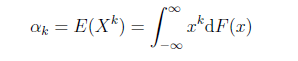R程序：计算S(1,2,3,4,5)的一阶原点矩(均值)

``````
> S<-c(1,2,3,4,5)
> mean(S)
 3
``````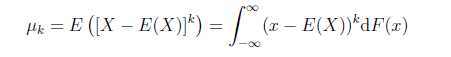R程序：计算S(1,2,3,4,5)的二阶中心矩(方差)

``````
> S<-c(1,2,3,4,5)
> var(S)
 2.5
``````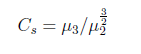``````
> library(PerformanceAnalytics)
> S<-rnorm(10000)
> skewness(S)
 -0.00178084
> hist(S,breaks=100)
``````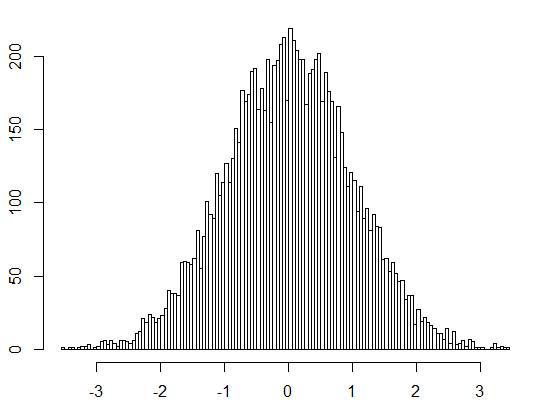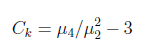R语言：计算10000个正态分布的样本的峰度，(同偏度的样本数据)

``````
> library(PerformanceAnalytics)
> kurtosis(S)
 -0.02443549
> hist(S,breaks=100)
``````

8). 协方差矩阵(covariance matrix)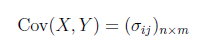R语言：计算协方差矩阵

``````
> x=as.data.frame(matrix(rnorm(10),ncol=2))
> x
V1          V2
1 -2.11315384 -2.55189840
2 -0.96631271 -1.36148355
3 -0.02835058 -0.82328774
4 -1.86669567 -0.07201353
5  0.27324957 -2.23835218

> var(x)
V1          V2
V1  1.13470650 -0.09292042
V2 -0.09292042  1.03172261

> cov(x)
V1          V2
V1  1.13470650 -0.09292042
V2 -0.09292042  1.03172261
``````

## 3. 极限定理

• 大数定律
• 中心极限定理

1). 大数定律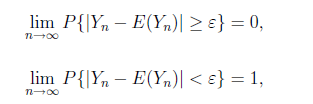• Bernoulli大数定律
• Chebyshev(切比雪夫)大数定律
• Khintchin(辛钦)大数定律

Bernoulli(贝努力)大数定律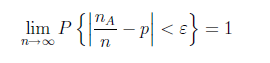Bernoulli大数定律揭示了“频率稳定于概率”说法的实质。

Chebyshev(切比雪夫)大数定律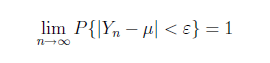Khintchin(辛钦)大数定律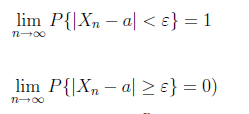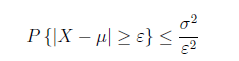R语言：假设投硬币，正面概率是0.5，投4次时，计算得到2次正面的概率？根据大数定律，如果投是10000次，计算5000次正面的概率？

``````
#计算2次正面的的概率
> choose(4,2)/2^4 #choose组合数的计算：从4中选择2个
 0.375

#计算5000次正面的的概率
> pbinom(5000, 10000, 0.5) #pbinom二向分布，5000为分位数，产生10000个随机数，每个概率0.5
 0.5039893
``````

2). 中心极限定理(central limit theorem)

• 列维定理(Lindburg-Levy)
• 拉普拉斯定理(de Movire - Laplace)

R语言：中心极限定理模拟，从指数分布到正态分布

``````
if (!require(animation)) install.packages("animation")
library(animation)
ani.options(interval = 0.1, nmax = 100)
par(mar = c(4, 4, 1, 0.5))
clt.ani()
``````